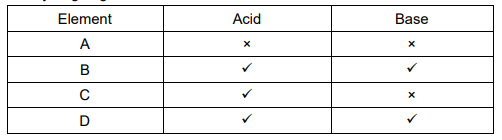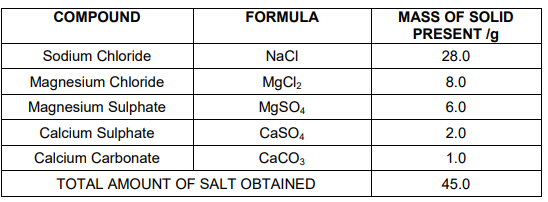# Acids, Bases and Salts Class 10 MCQ with answers

In this page we have around 40 mcq of acid base and salts class 10 are available for free along with a downloadable pdf of the questions. Acids, Bases, and Salts in CBSE Class 10 Science MCQs contain questions of varied difficulty levels to assist students in preparing for the board test. They can easily get the CBSE Class 10 Science MCQs Chapter 2 Acids Bases and Salts PDF by clicking on the link provided below. They can also obtain CBSE Class 10 Science MCQs for other chapters to help them prepare for the test.

The Acids, Bases, and Salts Class 10 MCQs have been created in accordance with the CBSE Class 10 Science Syllabus and cover the key concepts of Chapter 2. Practising these Class 10 MCQs on Acid Bases and Salts can improve students' preparation so that they may take the board exam with confidence. Students can also practise with the CBSE Class 10 Science Sample Papers based on the most recent exam pattern.

## Acids, bases and salts class 10 mcq

Question 1
When a solution of an acid contains larger amount of acid, it is said to be-
(a) Dilute
(b) concentrated
(c) Mono basic
(d) poly-basic

Question 2
Metals like sodium, potassium and calcium react with an acid to liberate-
(a) CO2
(b) NH3
(c) O2
(d) H2

Question 3
Which of the following is a weak acid?
(a) sulphuric acid
(b) hydrochloric acid
(c) acetic acid
(d) nitric acid

Question 4
The chemical formula of baking soda is
(a) Na2Co3
(b) Na2Co3.10H2O
(c) NaHCO3
(d) NaOH

Question 5
Which of the following phenomena occur, when a small amount of acid is added to water?
(a) ionization
(b) Neutralization
(c) Dilution
(d) Salt formation

Question 6
Salt form during reaction of sulphuric acid with copper
(a) Na2SO4
(b) CuSO4
(c) K2SO4
(d) NH4CI

Question 7
CuSO4. K2SO4   is
(a) acid salt
(b) a mixed salt
(c) normal salt
(d) double salt

Question 8
The acid present in vinegar
(a) citric acid
(b) tartaric acid
(c) ascorbic acid
(d) acetic acid

Question 9
Acid reacts with ____ of metals to form salt and water.
(a) hydroxide
(b) carbonates
(c) oxides
(d) sulphides

Question 10
Which of these bases is not an alkali?
(a) NaOH
(b) NH4OH
(c) Al (OH)3
(d) all of the are alkalies

Question 11
Which substance is used to purify water
(a) Ammonium chloride
(b) copper sulphate
(c) chlorine
(d) none of these

Question 12
H3PO4 is the example of-
(a) hydracids
(b) organic acid
(c) oxyacid
(d) salt

Question 13
To protect tooth decay we are advised to brush our teeth regularly. The nature of the tooth paste commonly used is
(a) acidic
(b) neutral
(c) basic
(d) corrosive

Question 14
Which of the following substance will not give carbon dioxide on treatment with dilute acid?
(a) Marble
(b) Limestone
(c) Baking soda
(d) Lime

Question 15
The reaction between an acid and a base to form a salt and water is called ________ reaction.
(a) neutralization
(b) endothermic
(c) both a and b
(d) none of these

Question 16
An indicator is what type of compound?
(a) reducing agent
(b) strong base or acid
(c) weak base or acid
(d) salt

Question 17
H2SO4 can be prepared by reaction of water with-
(a) NO2
(b) SO2
(c) N2O
(d) SO3

Question 18
The acidity of Fe(OH)3 is
(a) 2
(b) 3
(c) 4
(d) 5

Question 19
The example of olfactory indicators is
(a) Methyl orange
(b) onion
(c) blue litmus
(d) phenolphthalein

Question 20
The aqueous solution of sodium acetate is
(a) basic
(b) neutral
(c) acidic
(d) none of these

Question 21
Bleaching powder is soluble in cold water giving a milky solution due to
(a) Available chlorine
(b) Lime present in it
(c) Calcium carbonate formation
(d) The absorption of carbon dioxide from atmosphere

Question 22
A blue litmus paper was first dipped in dilute $HCl$ and then in dilute $NaOH$ solution. It was observed that the color of the litmus paper
(a) Changed to red
(b) Changed first to red and then to blue
(c) Changed blue to colorless
(d) Remains blue in both the solutions

Question 23
The acid used in making of vinegar is
(a) Formic acid
(b) Acetic acid
(c) Sulphuric acid
(d) Nitric acid

Question 24
A solution turns blue litmus red. The PH of the solution is probably
(a) 8
(b) 10
(c) 12
(d) 6

Question 25
Reaction of an acid with a base is known as
(a) decomposition
(b) combination
(c) redox reaction
(d) neutralization

Question 26
Antacids contains
(a) weak base
(b) weak acid
(c) strong base
(d) strong acid

Question 27
Bleaching powder gives smell of chlorine because
(a) is unstable
(b) gives chlorine on exposure to atmosphere
(c) is a mixture of chlorine and slaked lime
(d) contains excess of chlorine

Question 28
Plaster of paris is made from
(a) lime stone
(b) slaked lime
(c) quick lime
(d) gypsum

Question 29
Plaster of Paris hardens by
(a)giving of CO2
(b)changing into CaCO3
(c)combining with water
(d)giving out water

Question 30
Which of the following is acidic in nature
(a) apple juice
(b) soap solution
(c) slaked lime
(d) lime

Question 31
Which of the following acid is present in sour milk ?
(a) glycolic acid
(b) lactic acid
(c) citrus acid
(d) tartaric acid

Question 32
Milk of magnesium is an example of
(a) base
(b) acid
(c) salt
(d) none of these

Question 33
A solution react with crushed egg- shells to give a gas that turns lime- water milky. The solution contains
(a) NaCl
(b) HCl
(c) LiCl
(d) KCl

Question 34
Which of the following is the weak acid
(a) sulphuric acid
(b) hydrochloric acid
(c) acetic acid
(d) nitric acid

Question 35
Acidity of ammonium hydroxide is
(a) 1
(b) 2
(c) 4
(d) 3

Question 36
Which of the following is not an acidic salt
(a)CuSO4
(b)Na2CO3
(c)ZnSO4
(d)NH4NO3

Question 37
Basic salts are formed by neutralization of
(a)strong acid and strong base
(b)strong acid and weak base
(c)weak acid and weak base
(d)strong base and weak acid

Question 38
Plaster of paris is obtained
(a)by adding water to calcium sulphate
(b)by adding sulphuric acid to calcium hydroxide
(c)by heating gypsum to a very high temperature
(d)by heating gypsum to 373 K.

Question 39
When we heat the carbonates of some metals, we get
(a) SO2
(b) H2
(c) CO2
(d) NO2

Question 40
The chemical formula of caustic potash is
(a) $NaOH$
(b) $Ca(OH)_2$
(c) $NH_4OH$
(d) $KOH$

Question 41
The table given below shows the reaction of a few elements with acids and bases to evolve Hydrogen gas.(a) A and D
(b) B and D
(c) A and C
(d) C and D

Case Study question
1. The salt pans in Marakkanam, a port town about 120 km from Chennai are the third largest producer of salt in Tamil Nadu. Separation of salt from water is a laborious process and the salt obtained is used as raw materials for manufacture of various sodium compounds.
2. One such compound is Sodium hydrogen carbonate, used in baking, as an antacid and in soda acid fire extinguishers.
3. The table shows the mass of various compounds obtained when 1 litre of sea water is evaporatedQuestion 42
Which compound in the table reacts with acids to release carbon dioxide?
(a)NaCl
(b)CaSO4
(c)CaCO3
(d)MgSO4

Question 43
How many grams of Magnesium Sulphate are present in 180g of solid left by evaporation of sea water?
(a)12g
(b)6g
(c)24g
(d)18g

Question 44
What is the saturated solution of Sodium Chloride called?
(a)Brine
(b)Slaked lime
(c)Soda water
(d)Lime water

Question 45
What is the pH of the acid which is used in the formation of common salt??
(a)Between 1 and 3
(b)Between 3 and 7
(c)Between 7 and 11
(d)Between 7 and 14